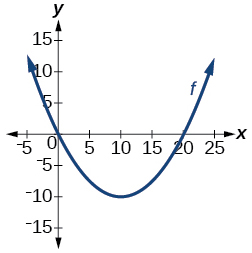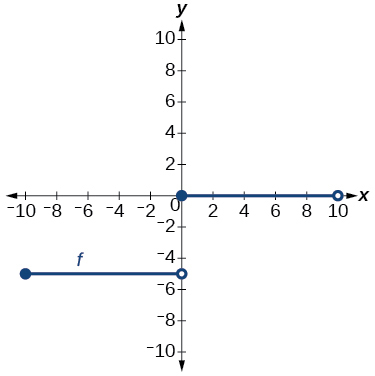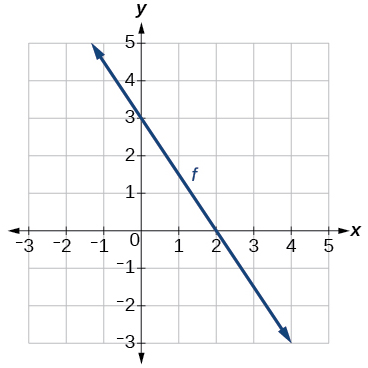# 1.7E: Exercises

$$\newcommand{\vecs}{\overset { \rightharpoonup} {\mathbf{#1}} }$$ $$\newcommand{\vecd}{\overset{-\!-\!\rightharpoonup}{\vphantom{a}\smash {#1}}}$$$$\newcommand{\id}{\mathrm{id}}$$ $$\newcommand{\Span}{\mathrm{span}}$$ $$\newcommand{\kernel}{\mathrm{null}\,}$$ $$\newcommand{\range}{\mathrm{range}\,}$$ $$\newcommand{\RealPart}{\mathrm{Re}}$$ $$\newcommand{\ImaginaryPart}{\mathrm{Im}}$$ $$\newcommand{\Argument}{\mathrm{Arg}}$$ $$\newcommand{\norm}{\| #1 \|}$$ $$\newcommand{\inner}{\langle #1, #2 \rangle}$$ $$\newcommand{\Span}{\mathrm{span}}$$ $$\newcommand{\id}{\mathrm{id}}$$ $$\newcommand{\Span}{\mathrm{span}}$$ $$\newcommand{\kernel}{\mathrm{null}\,}$$ $$\newcommand{\range}{\mathrm{range}\,}$$ $$\newcommand{\RealPart}{\mathrm{Re}}$$ $$\newcommand{\ImaginaryPart}{\mathrm{Im}}$$ $$\newcommand{\Argument}{\mathrm{Arg}}$$ $$\newcommand{\norm}{\| #1 \|}$$ $$\newcommand{\inner}{\langle #1, #2 \rangle}$$ $$\newcommand{\Span}{\mathrm{span}}$$$$\newcommand{\AA}{\unicode[.8,0]{x212B}}$$

## Section Exercises

Verbal

Exercise 1.7.1

Describe why the horizontal line test is an effective way to determine whether a function is one-to-one?

Each output of a function must have exactly one output for the function to be one-to-one. If any horizontal line crosses the graph of a function more than once, that means that $$y$$-values repeat and the function is not one-to-one. If no horizontal line crosses the graph of the function more than once, then no $$y$$-values repeat and the function is one-to-one.

Exercise 1.7.2

Why do we restrict the domain of the function $$f(x)=x^2$$ to find the function’s inverse?

Exercise 1.7.3

Can a function be its own inverse? Explain.

Yes. For example, $$f(x)=\frac{1}{x}$$ is its own inverse.

Exercise 1.7.4

Are one-to-one functions either always increasing or always decreasing? Why or why not?

Exercise 1.7.5

How do you find the inverse of a function algebraically?

Given a function $$y=f(x)$$, solve for $$x$$ in terms of $$y$$. Interchange the $$x$$ and $$y$$. Solve the new equation for $$y$$. The expression for $$y$$ is the inverse, $$y=f^{-1}(x)$$.

Algebraic

Exercise 1.7.6

Show that the function $$f(x)=a−x$$ is its own inverse for all real numbers $$a$$.

For the following exercises, find $$f^{-1}(x)$$ for each function.

Exercise 1.7.7

$$f(x)=x+3$$

$$f^{-1}(x)=x−3$$

Exercise 1.7.8

$$f(x)=x+5$$

Exercise 1.7.9

$$f(x)=2−x$$

$$f^{-1}(x)=2−x$$

Exercise 1.7.10:

$$f(x)=3−x$$

Exercise 1.7.11

$$f(x)=\frac{x}{x+2}$$

$$f^{-1}(x)=\frac{−2x}{x−1}$$

Exercise 1.7.12

$$f(x)=\frac{2x+3}{5x+4}$$

For the following exercises, find a domain on which each function $$f$$ is one-to-one and non-decreasing. Write the domain in interval notation. Then find the inverse of $$f$$ restricted to that domain.

Exercise 1.7.13

$$f(x)=(x+7)^2$$

domain of $$f(x)$$: $$\left[−7,\infty\right)$$; $$f^{-1}(x)=\sqrt{x}−7$$

Exercise 1.7.14

$$f(x)=(x−6)^2$$

Exercise 1.7.15

$$f(x)=x^2−5$$

domain of $$f(x)$$: $$\left[0,\infty\right)$$; $$f^{-1}(x)=\sqrt{x+5}$$

Exercise 1.7.16

Given $$f(x)=\frac{x}{2+x}$$ and $$g(x)=\frac{2x}{1-x}:$$

a. Find $$f(g(x))$$ and $$g(f(x))$$.

b. What does the answer tell us about the relationship between $$f(x)$$ and $$g(x)?$$

a. $$f(g(x))=x$$ and $$g(f(x))=x$$.

b. This tells us that $$f$$ and $$g$$ are inverse functions

For the following exercises, use function composition to verify that $$f(x)$$ and $$g(x)$$ are inverse functions.

Exercise 1.7.17

$$f(x)=\sqrt{x-1}$$ and $$g(x)=x^3+1$$

$$f(g(x))=x$$, $$g(f(x))=x$$

Exercise 1.7.18

$$f(x)=−3x+5$$ and $$g(x)=\frac{x-5}{-3}$$

Graphical

For the following exercises, use a graphing utility to determine whether each function is one-to-one.

Exercise 1.7.19

$$f(x)=\sqrt{x}$$

one-to-one

Exercise 1.7.20

$$f(x)=\sqrt{3x+1}$$

Exercise 1.7.21

$$f(x)=−5x+1$$

one-to-one

Exercise 1.7.22

$$f(x)=x^3−27$$

For the following exercises, determine whether the graph represents a one-to-one function.

Exercise 1.7.23not one-to-one

Exercise 1.7.24For the following exercises, use the graph of $$f$$ shown in Figure 1.7.11.Figure 1.7.11: Graph of a line

Exercise 1.7.25

Find $$f(0)$$.

3

Exercise 1.7.26

Solve $$f(x)=0$$.

Exercise 1.7.27

Find $$f^{-1}(0)$$.

2

Exercise 1.7.28

Solve $$f^{-1}(x)=0$$.

For the following exercises, use the graph of the one-to-one function shown in Figure 1.7.12.Figure 1.7.12: Graph of a square root function.

Exercise 1.7.29

Sketch the graph of $$f^{-1}$$.Exercise 1.7.30

Find $$f(6)$$ and $$f^{-1}(2)$$.

Exercise 1.7.31

If the complete graph of $$f$$ is shown, find the domain of $$f$$.

$$[2,10]$$

Exercise 1.7.32

If the complete graph of $$f$$ is shown, find the range of $$f$$

Numeric

For the following exercises, evaluate or solve, assuming that the function $$f$$ is one-to-one.

Exercise 1.7.33

If $$f(6)=7$$, find $$f^{-1}(7)$$.

6

Exercise 1.7.34

If $$f(3)=2$$, find $$f^{-1}(2)$$.

Exercise 1.7.35

If $$f^{-1}(−4)=−8$$, find $$f(−8)$$.

-4

Exercise 1.7.36

If $$f^{-1}(−2)$$=−1, find $$f(−1)$$.

For the following exercises, use the values listed in Table 1.7.6 to evaluate or solve.

 $$x$$ $$f(x)$$ 0 8 1 0 2 7 3 4 4 2 5 6 6 5 7 3 8 9 9 1

Table 1.7.6

Exercise 1.7.37

Find $$f(1)$$.

0

Exercise 1.7.38

Solve $$f(x)=3$$.

Exercise 1.7.39

Find $$f^{-1}(0)$$.

1

Exercise 1.7.40

Solve $$f^{-1}(x)=7$$.

Exercise 1.7.41

Use the tabular representation of $$f$$ in Table 1.7.7 to create a table for f^{-1}(x).

 $$x$$ $$f(x)$$ 3 6 9 13 14 1 4 7 12 16

Table 1.7.7

 $$x$$ $$f^{-1}(x)$$ 1 4 7 12 16 3 6 9 13 14

Technology

For the following exercises, find the inverse function. Then, graph the function and its inverse.

Exercise 1.7.42

$$f(x)=\dfrac{3}{x-2}$$

Exercise 1.7.43

$$f(x)=x^3−1$$

$$f^{-1}(x)=(1+x)^{1/3}$$Exercise 1.7.44

Find the inverse function of $$f(x)=\frac{1}{x-1}$$. Use a graphing utility to find its domain and range. Write the domain and range in interval notation.

Real-World Applications

Exercise 1.7.45

To convert from $$x$$ degrees Celsius to $$y$$ degrees Fahrenheit, we use the formula $$f(x)=\frac{9}{5}x+32$$. Find the inverse function, if it exists, and explain its meaning.

$$f^{-1}(x)=\frac{5}{9}(x−32)$$. Given the Fahrenheit temperature, $$x$$, this formula allows you to calculate the Celsius temperature.

Exercise 1.7.46

The circumference $$C$$ of a circle is a function of its radius given by $$C(r)=2{\pi}r$$. Express the radius of a circle as a function of its circumference. Call this function $$r(C)$$. Find $$r(36\pi)$$ and interpret its meaning.

Exercise 1.7.47

A car travels at a constant speed of 50 miles per hour. The distance the car travels in miles is a function of time, $$t$$, in hours given by $$d(t)=50t$$. Find the inverse function by expressing the time of travel in terms of the distance traveled. Call this function $$t(d)$$. Find $$t(180)$$ and interpret its meaning.

$$t(d)=\frac{d}{50}$$, $$t(180)=\frac{180}{50}$$. The time for the car to travel 180 miles is 3.6 hours.